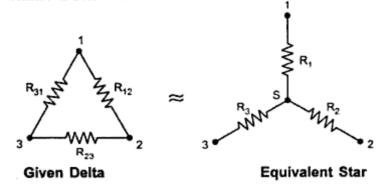### Delta Star Transformation

Consider the three resistances R12  , R23  ,R31  connected in delta as shown in the Fig.1. The terminals between which are these connected in Delta are named as 1, 2 and 3.Fig.  1
Now it is always possible to replace these Delta connected resistances by three equivalent Star connected resistances R1, R , R between the same terminals 1, 2, and 3. Such a star is shown inside the Delta in the Fig. 1 which is called equivalent Star of  Delta connected resistances.
Key point : Now to call these tow arrangements as equivalent, the resistance between any tow terminals must be same in both the types of connections.
Let us analyse Delta connection first, shown in the Fig. 2(a).Fig.  2
Now consider the terminals (1) and (2). Let us find equivalent resistance between (1) and (2). We can redraw the network as viewed from the terminals (1) and (2), without considering terminal (3). This is shown in the Fig. 2(b).
Now terminal '3' we are not considering , so between terminal (1)  and (2) we get the combination as,
R12   parallel with ( R31 + R23 ) as R31 and R23 are in series.
Between (1) and (2) the resistance is,
{using (R1 R2 )/(R1 +R2 ) for parallel combination)
Now consider the same tow terminals of equivalent Star connection shown in the Fig. 3.

Now as viewed from terminals (1) and (2) we see that terminal (3) is not getting connected anywhere and hence is not playing any role in deciding the resistances viewed from terminals (1) and (2).
And hence we can redraw the network as viewed through the terminals (1) and (2) as shown in the Fig. 4.
...        Between (1) and (2) the resistance is = R1 + R2           .................... (b)
This is because, tow of them found to be in series across the terminals 1 and 2 while 3 found to be open.
Now to call this Star as equivalent of given Delta it is necessary that the resistances calculated between terminals (1) and (2) in both the cases should be equal and hence equating equations (a) and (b),
Similarly if we find the equivalent resistance as viewed through terminals (2) and (3) in both the cases and equating, we get,
Similarly if we find the equivalent resistance as viewed through terminals (3) and (1) in both the cases and equating, we get,
Now we are interested in calculating what are the values of R1 ,R2 ,R interms of known values  R12 ,  R23 , andR31.
Subtracting equation (f) and equation (c),
Adding equation (f) and equation (e),
Similarly by using another combinations of subtraction and addition with equations (c), (d) and (e) we can get,
and

Easy way for remembering the result :
The equivalent star resistance between any terminal and star point is equal to the product of the tow resistances in delta, which are connected to same terminal, divided by the sum of all three delta connected resistances Fig.  5.
So if we want equivalent resistance between terminal (2) and star point i.e. R2 then it is the product of tow resistances in delta which are connected to same terminal i.e. terminal (2) which are  R12 and R23 divided by sum of all delta connected resistances i.e. R12 ,R23 and R31.Fig .  5
Note : If all resistances in a Delta connection have same magnitude say R, then its equivalent Star will contain,
R1 = R2 = R3 = ( R x R)/(R + R + R) =R/3